## Social Question# Do you agree with Keith Devlin that multiplication should not be taught as repeated addition?

Asked by LostInParadise (29140) April 13th, 2012

Keith Devlin is a mathematician who has written a number of pop math books and is NPR’s “math guy.” I usually enjoy following his work, but I disagree fairly strongly with the idea expressed here

My feeling is that, as much as possible, teachers should make use of students’ intuitive grasp of the material. Thinking of multiplication as repeated addition makes it easier to understand some basic arithmetic properties. For example, to show that a*b = b*a, arrange items it rows and columns and then rotate the collection by 90 degrees to show rows and columns exchange roles, but the total remains the same. To teach the distributive law, look at 5(3+4) as (3+4) + (3+4) +(3+4) +(3+4) +(3+4) = 5*3 + 5*4.

In a similar way, exponentiation is easier to understand if it is first introduced as repeated multiplication. (a^b)(a^c) = a^(b+c) makes perfectly good sense if you think of combining b multiplications of a with c multiplications.

Historically, whole numbers were understood before fractions and decimals. It is certainly not necessary to teach things in historical sequence, but the history does provide a guide to the order in which understanding can be achieved. It seems perfectly natural to me to look at the arithmetic of real numbers as an extension of the arithmetic of whole numbers.

Observing members: 0Composing members: 0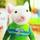I think multiplication can be more easily understood if students are taught to look at multiplication tables as a pattern. I struggled with multiplication until I took my first algebra class when I returned to school as a grown up. The very first time I looked at multiplication I saw that it was a repeating pattern and from that point forward I could recite all of my multiplication tables without a second thought. As someone who is not a math person I found the whole idea of “repeated addition” very confusing and it did not help me learn my multiplication tables. All three of my children were taught to multiply using the repeated addition technique and all three of them struggled. We are currently working with the youngest to help him and using the idea of patterns to get the job done. So yes, I agree that teaching multiplication as repeated addition is not a great idea.

SuperMouse (30837)“Great Answer” (1) Flag as…@SuperMouse, Your experience suggests that the idea of repeated addition does not make things any clearer and is in fact an impediment to learning multiplication. This goes against my intuitive feel for the subject, but in teaching you got to go with what works.

LostInParadise (29140)“Great Answer” (0) Flag as…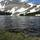Different methods work for different people. I always had problems with math, and learning different ways helped me understand.

Blackberry (31933)“Great Answer” (3) Flag as…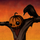A philosophical point:

The linked article is at risk of being internally inconsistent. The grounds on which he rejects subtraction and division as basic operations can be generalized, making his insistence that multiplication is basic more tenuous (as well as undermining his point about exponentiation). He rejects subtraction and division as basic because they are the inverses of addition and multiplication, respectively. He also acknowledges that multiplication and repeated addition give you the same results for natural numbers.

Having said all this, he attacks the notion that multiplication is repeated addition through an argument about fractions. Unfortunately, that argument can be countered using only that which he has already admitted. Give someone addition and the natural numbers, and his views about what is basic get you subtraction (since it’s “just” the inverse of addition) and the set of integers (since we can get these from the natural numbers plus subtraction—we even get to bypass debates over whether or not 0 is a natural number).

Once we have all this, we can explain the multiplication of fractions by breaking them down into a series of additive relationships among integers. We simply need to treat the numerator and the denominator separately, acting as if we were doing two different equations. Moreover, this separation of the numerator and the denominator is precisely how the multiplication of fractions is often introduced to students. Perhaps Devlin doesn’t like this either, though I address that in the next section. The point for now is just that his argument is unstable. He would do better to focus on the differences between multiplication and summation, and to give up his rejection of subtraction and division as basic (which I notice he does do in later blog entries).

An educational point:

It is true that multiplication is not repeated addition. Multiplication is multiplication. It concerns scalar relationships, not additive ones. Education, however, is an additive relationship: you teach something new by building on what the students already know. So while Mr. Devlin may be correct that teachers should not just blithely say that multiplication is repeated addition, there is no reason why they should not point out the similarity that a great deal of multiplication problems have to repeated addition when teaching it.

Note that the linked article is actually a bit of a paper tiger: it ends by basically admitting precisely what I’ve just said. Mr. Devlin acknowledges that he is making a fairly technical point about mathematics and that he does not know exactly how it should translate into the field of education. What I will say, then, is simply this: a good teacher has several different ways of explaining any given point. Thus it would be counterproductive to not have the “multiplication as repeated addition” line in one’s bag of tricks. “Multiplication as repeated addition,” however, is different from “multiplication is repeated addition.” The former only rests on using the similarities to prompt initial understanding. It is hard to see how Mr. Devlin could reasonably object to this.

I take it that this is the point @Blackberry ended up making while I was typing this.

SavoirFaire (27761)“Great Answer” (2) Flag as…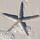I do not see a problem with teaching it that way.

marinelife (62470)“Great Answer” (2) Flag as…Here is a follow-up article, a bit longish. In the article, there is an extended analogy comparing going from whole numbers to real numbers to going from horse and buggy to car. There is a big difference between the two situations. A person in the modern world can live quite successfully without ever knowing anything about a horse and buggy. However, in learning about numbers, we start at a very young age with whole numbers when we learn to count. It may be possible to teach real numbers without first introducing counting, but it would sure be one tough process.

Devlin talks about the modern abstract algebra structures that can be applied to numbers, but he fails to mention that in fact real numbers can be derived axiomatically in pretty much the way that we are taught in school. All of the arithmetic of whole numbers can be developed from the idea of counting. For anyone interested in this, you can look into the Peano axioms. There are ways of then defining negative numbers in terms of whole numbers, and rational numbers in terms of integers, and finally real numbers in terms of rational numbers.

In Peano’s develpment of whole number arithmetic, he defines addition in terms of counting and then defines multiplication in terms of addition. Devlin discusses this here Even without fully understanding how the axioms work, you can see from the definitions of P, which is addition and M, which is multiplication, that P is defined in terms of S, which is essentially the process of counting, and M is defined in terms of P and S. It is perfectly reasonable to think of the recursion embodied in the definitions as a way of talking about repeated operations. Therefore, even in the most mathematically abstract sense, multiplication is defined as repeated addition.

LostInParadise (29140)“Great Answer” (1) Flag as…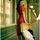I used a multiplication table. It was mostly memorization, but that has worked just fine for about 3 decades.

Supacase (14543)“Great Answer” (0) Flag as…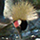I drives me bonkers that they don’t teach all of these operations as being about things. 4 packages of cookies, each with 3 cookies is them is 12 cookies (aka lunch)

It looks like this

####
####
####

That’s what 4 times 3 is. It’s a description of something, not an algorithm on how to calculate the answer.

6rant6 (13692)“Great Answer” (2) Flag as…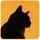I taught third grade for years and in the first week I had each student bring in a box of 100 straws. When teaching the beginnings of multiplication I had them arranging things in equal groups and got them in the habit of saying, for example, three times two is three groups of two. Added together they make a group of six, and so forth.

Rather than simply having to rote memorize tables, I wanted to be certain that they understood precisely what those numbers represented in a concrete way. There was plenty of time during the whole rest of the year to focus on memorization since I gave a quick ten question quiz each day.

Was that just hopelessly old-fashioned? I don’t know and I didn’t really care because I felt that the concrete manipulation of actual objects ensured that the thorough understanding of the basic concepts was firmly in place so that the need for all the tedious memorization to follow was obvious. It didn’t take too long for them to realize that its a lot quicker to remember all these groups of numbers in relational patterns than to laboriously count stuff out each time. You really don’t want to have to spend life dragging a box of straws around with you :)

Of course, nowadays everybody has a calculator function on their phone, so that’s kind of a moot point; but that wasn’t an option till recently. But if I were still teaching third grade today, I would likely still employ the box of straws for the first month or so. It worked remarkably well and I’m a pragmatist.

Buttonstc (27597)“Great Answer” (2) Flag as…